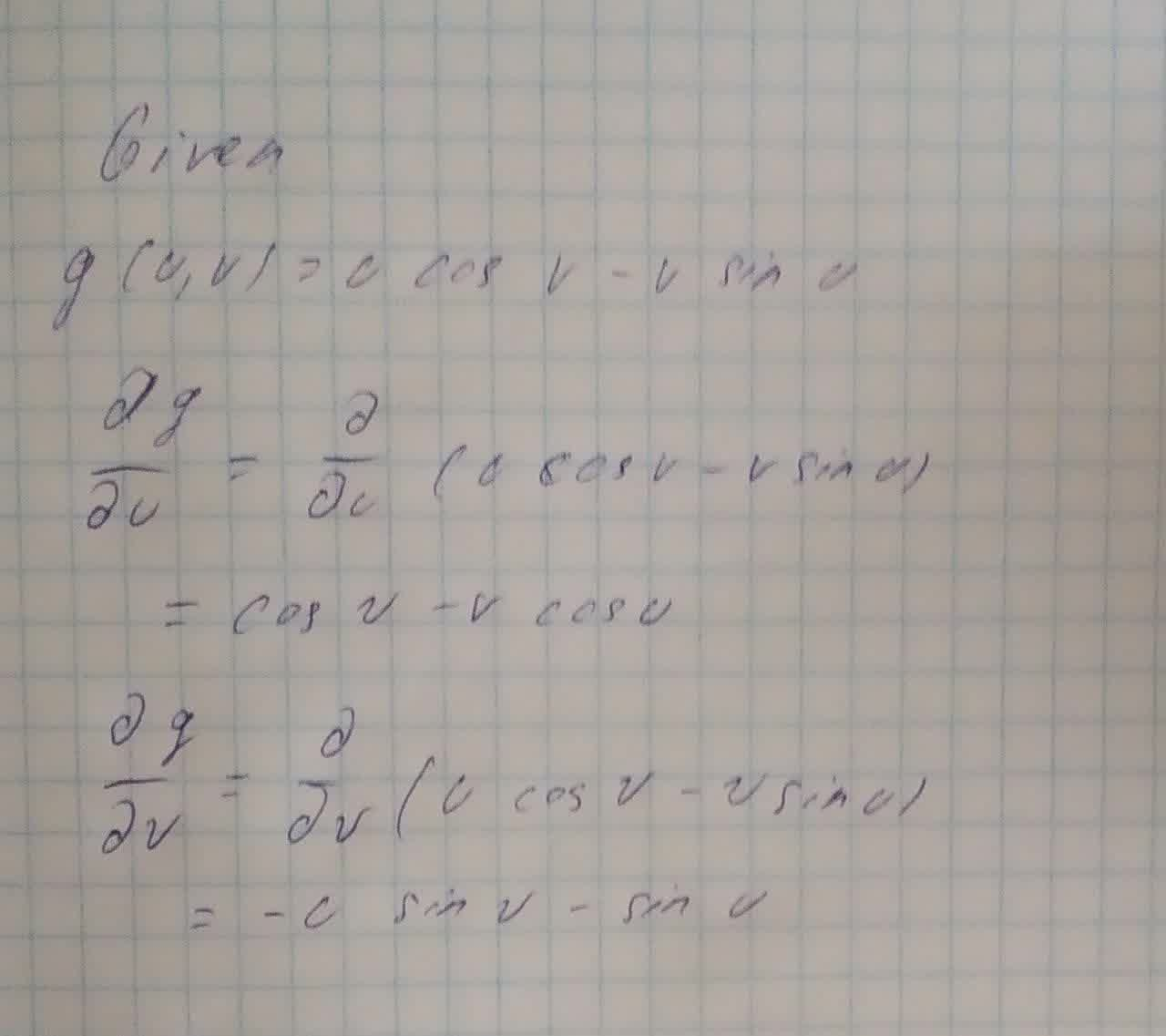Question# Find the first partial derivatives of the following functions. g(u,v)=u\cos v-v\sin

Derivatives
ANSWEREDFind the first partial derivatives of the following functions.
$$\displaystyle{g{{\left({u},{v}\right)}}}={u}{\cos{{v}}}-{v}{\sin{{u}}}$$